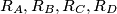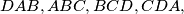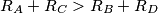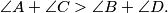### IMO Shortlist 1996 problem G8

Kvaliteta:
Avg: 0,0
Težina:
Avg: 9,0
Let$ABCD$ be a convex quadrilateral, and let$R_A, R_B, R_C, R_D$ denote the circumradii of the triangles$DAB, ABC, BCD, CDA,$ respectively. Prove that$R_A + R_C > R_B + R_D$ if and only if$\angle A + \angle C > \angle B + \angle D.$
Izvor: Međunarodna matematička olimpijada, shortlist 1996